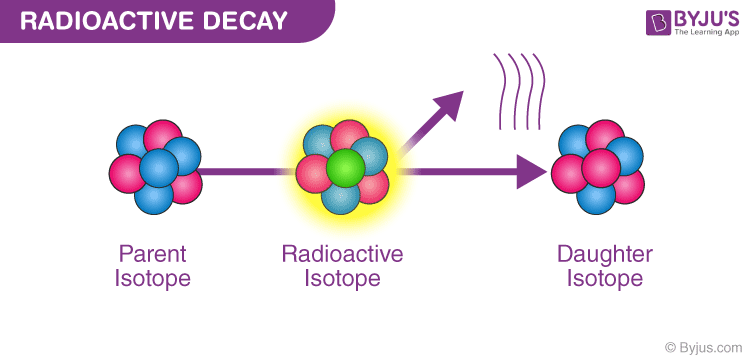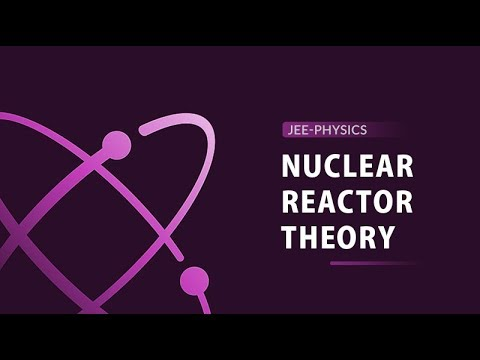Many significant activities in nature take place around us and are unnoticed. Emission and absorption are some of the phenomena that go unnoticed when it takes place in the atom. Radioactivity is one such property of the matter where the emission of energetic subatomic particles takes place spontaneously. In this article, let us learn more about what radioactivity is.

Due to nuclear instability, an atom’s nucleus exhibits the phenomenon of Radioactivity. Energy is lost due to radiation that is emitted out of the unstable nucleus of an atom. Two forces, namely the force of repulsion that is electrostatic and the powerful forces of attraction of the nucleus, keep the nucleus together. These two forces are considered extremely strong in the natural environment. The chance of encountering instability increases as the size of the nucleus increases because the mass of the nucleus becomes a lot when concentrated. That’s the reason why atoms of Plutonium, Uranium are extremely unstable and undergo the phenomenon of radioactivity.

Henry Becquerel discovered radioactivity by accident. A Uranium compound was placed in a drawer containing photographic plates, wrapped in a black paper. When the plates were examined later, it was found that they were exposed! This exposure gave rise to the concept of Radioactive decay. Radioactivity can be seen in such forms

• Gamma Decay (Photons having high energy are emitted)
• Beta Decay (Emission consists of Electrons)
• Alpha Decay (Emission consists of Helium nucleus)

Following are the three radioactive radiations that are obtained from α, β, and γ rays:

 $$\begin{array}{l}X_{z}^{a}(mother\;nucleus)\rightarrow Y_{z-2}^{a-4}(daughter\;nucleus)+He_{2}^{4}(\alpha \;particle)\end{array}$$ $$\begin{array}{l}X_{z}^{a}(mother\;nucleus)\rightarrow Y_{z+1}^{a}(daughter\;nucleus)+e_{-1}^{0}(radiation)\end{array}$$ $$\begin{array}{l}X_{z}^{+a}(mother\;nucleus)\rightarrow Y_{z}^{a}(daughter\;nucleus)+h_{f}(\gamma \;ray)\end{array}$$

After understanding what is radioactivity, let us now learn the laws of radioactivity.

• Radioactivity is the result of the decay of the nucleus.
• The nucleus’s decay rate is independent of temperature and pressure.
• Radioactivity is dependent on the law of conservation of charge.
• The physical and chemical properties of the daughter nucleus are different from the mother nucleus.
• The emission of energy from radioactivity is always accompanied by alpha, beta, and gamma particles.
• The rate of decay of radioactive substances is dependent on the number of atoms that are present at the time.Curie and Rutherford are the units of radioactivity.

1C = 3.7 × 104 Rd is the relationship between Curie and Rutherford.

## Alpha Decay

Alpha decay is a type of radioactive decay where the unstable atomic nuclei emit a helium nucleus (alpha particle) and in the process transforms into another more stable element. The particle ejected out, the alpha particle, consists of four nucleons, and they are two neutrons and two protons. Alpha radiation reduces the ratio of protons to neutrons in the parent nucleus, bringing it to a more stable configuration. An alpha particle is identical to the nucleus of a helium atom. The first observations and investigation into alpha decay were made by Ernest Rutherford, who used alpha particles in his gold foil scattering experiment. As the alpha particle; made of two protons and neutron; exits the nucleus, the atomic number of the radioactive sample changes. The element left behind after alpha decay is two atomic numbers lesser and four mass numbers lower. For example, Uranium 238 92 decays to form Thorium23490.

$$\begin{array}{l}_{92}^{238}\textrm{U} \rightarrow _{90}^{234}\textrm{Th} + _{2}^{4}\textrm{He}\end{array}$$

Thus, the transformation of a nucleus in an alpha decay can be written as;

$$\begin{array}{l}_{Z}^{A}\textrm{X} \rightarrow _{Z-2}^{A-4}\textrm{Y} + _{2}^{4}\textrm{He}\end{array}$$

### Occurrence of alpha decay

Alpha decay occurs only in the heaviest of the elements. The element’s nucleus should be large or unstable enough to undergo spontaneous fission-type changes. It is the most common form of decay in such elements. The alpha particles emitted out of the nucleus usually have an energy level of around 5 MeV and have a speed of around 5% of light. It is important to note that alpha particles possess a charge of +2 due to the absence of electrons. Due to this charge and owing to its heavy mass, an alpha particle reacts with the surroundings vigorously to lose all of its energy almost immediately. Their forward motion can be stopped by a few centimeters of air.

Owing to their heaviness and their charge, this kind of radioactive decay reacts most violently with the human body. They have a high ionizing power due to which they can wreak havoc with a tissue. An overdose of alpha radiation results in the formation of blisters and burns on the victim’s bodies.

Some radioactivity uses are provided in the points below.

• Americium-241 is an alpha emitter and is used for domestic smoke detectors in the United States.
• The alpha particles given out by the Americium sample ionize the air in the chamber of the smoke detector leading to a small current in the chamber.
• When smoke enters the chamber, it causes a drop in current causing the alarm to go off. Although Alpha particles have a very short range, they are devastating when in close contact.
• Alpha emitters, when swallowed, come in close contact with tissue and are deadly in such circumstances and is therefore used in assassination attempts by radiation poisoning.• Gamma rays are used to kill cancerous cells and hence used in radiotherapy.
• Cobalt-60 is used to destroy carcinogenic cells.
• Gamma rays are used in scanning the internal parts of the body.
• Gamma rays kill microbes present in food and prevent it from decay by increasing the shelf life.
• Age of the rocks can be studied using radioactive radiations by measuring the argon content present in the rock.

In this article, you learned what is radioactivity. Want to know more? Join BYJU’S and fall in love with learning. Also, register to “BYJU’S – The Learning App” for loads of interactive, engaging Physics-related videos and unlimited academic assist.

## Frequently Asked Questions – FAQs

Q1

### What is meant by the half-life of an isotope?

The half-life of an isotope is the average time it takes for half of the atoms to decay.

Q2

### List a few uses of radioactivity.

Following are a few uses of radioactivity:

• Used in domestic smoke detectors
• Used to sterilize medical instruments
• Used to diagnose and treat diseases
• Used to produce electric power
Q3

Radioactivity is a phenomenon exhibited by a few matters of emitting energy and subatomic particles spontaneously. Radioactivity is due to the nuclear instability of an atom.

Q4